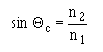Custom SearchBASIC OPTICAL-MATERIAL PROPERTIES The basic optical property of a material, relevant to optical fibers, is the index of refraction. The index of refraction (n) measures the speed of light in an optical medium. The index of refraction of a material is the ratio of the speed of light in a vacuum to the speed of light in the material itself. The speed of light (c) in free space (vacuum) is 3 X 108 meters per second (m/s). The speed of light is the frequency (f) of light multiplied by the wavelength of light (λ). When light enters the fiber material (an optically dense medium), the light travels slower at a speed (v). Light will always travel slower in the fiber material than in air. The index of refraction is given by:A light ray is reflected and refracted when it encounters the boundary between two different transparent mediums. For example, figure 2-7 shows what happens to the light ray when it encounters the interface between glass and air. The index of refraction for glass (n1) is 1.50. The index of refraction for air (n2) is 1.00. Figure 2-7. - Light reflection and refraction at a glass-air boundary.Let's assume the light ray or incident ray is traveling through the glass. When the light ray encounters the glass-air boundary, there are two results. The first result is that part of the ray is reflected back into the glass. The second result is that part of the ray is refracted (bent) as it enters the air. The bending of the light at the glass-air interface is the result of the difference between the index of refractions. Since n1 is greater than n2, the angle of refraction (Θ2) will be greater than the angle of incidence (Θ1). Snell's law of refraction is used to describe the relationship between the incident and the refracted rays at the boundary. Snell's Law is given by:As the angle of incidence (Θ1) becomes larger, the angle of refraction (Θ2) approaches 90 degrees. At this point, no refraction is possible. The light ray is totally reflected back into the glass medium. No light escapes into the air. This condition is called total internal reflection. The angle at which total internal reflection occurs is called the critical angle of incidence. The critical angle of incidence (Θc) is shown in figure 2-8. At any angle of incidence (Θ1) greater than the critical angle, light is totally reflected back into the glass medium. The critical angle of incidence is determined by using Snell's Law. The critical angle is given by:Figure 2-8. - Critical angle of incidence.The condition of total internal reflection is an ideal situation. However, in reality, there is always some light energy that penetrates the boundary. This situation is explained by the mode theory, or the electromagnetic wave theory, of light. Q.14 What is the basic optical-material property relevant to optical fiber light transmission? Q.15 The index of refraction measures the speed of light in an optical fiber. Will light travel faster in an optically dense material or in one that is less dense? Q.16 Assume light is traveling through glass, what happens when this light strikes the glass-air boundary? Q.17 What condition causes a light ray to be totally reflected back into its medium of propagation? Q.18 What name is given to the angle where total internal reflection occurs?Integrated Publishing, Inc. - A (SDVOSB) Service Disabled Veteran Owned Small Business